# Test: 35 Year JEE Previous Year Questions: Simple Harmonic Motion (Oscillations)

## 39 Questions MCQ Test Physics 35 Years JEE Main & Advanced Past year Papers | Test: 35 Year JEE Previous Year Questions: Simple Harmonic Motion (Oscillations)

Description
Attempt Test: 35 Year JEE Previous Year Questions: Simple Harmonic Motion (Oscillations) | 39 questions in 80 minutes | Mock test for JEE preparation | Free important questions MCQ to study Physics 35 Years JEE Main & Advanced Past year Papers for JEE Exam | Download free PDF with solutions
QUESTION: 1

### When a particle of mass m moves on the x-axis in a potential of the form V(x) = kx2 it performs simple harmonic motion. The corresponding time period is proportional to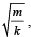as can be seen easily using dimensional analysis. However, the motion of a particle can be periodic even when its potential energy increases on both sides of x = 0 in a way different from kx2 and its total energy is such that the particle does not escape to infinity. Consider a particle of mass m moving on the x-axis. Its potential energy is V(x) = αx4 (α > 0) for |x| near the origin and becomes a constant equal to V0 for |x| > X0 (see figure).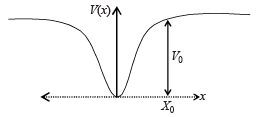Q. If the total energy of the particle is E, it will perform periodic motion only if

Solution:

If the energy is zero, the particle will not perform oscillations. Therefore E should be greater than zero.
Further if E = V0, the potential energy will become constant as depicted in the graph given. In this case also the particle will not oscillate.
Therefore E should be greater than zero but less than V0.

QUESTION: 2

### When a particle of mass m moves on the x-axis in a potential of the form V(x) = kx2 it performs simple harmonic motion. The corresponding time period is proportional toas can be seen easily using dimensional analysis. However, the motion of a particle can be periodic even when its potential energy increases on both sides of x = 0 in a way different from kx2 and its total energy is such that the particle does not escape to infinity. Consider a particle of mass m moving on the x-axis. Its potential energy is V(x) = αx4 (α > 0) for |x| near the origin and becomes a constant equal to V0 for |x| > X0 (see figure).Q. For periodic motion of small amplitude A, the time period T of this particle is proportional to

Solution:

We can get the answer of this question with the help of dimensional analysis.

Given potential energy = αx4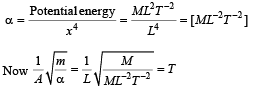Therefore option (b) is correct.

QUESTION: 3

### When a particle of mass m moves on the x-axis in a potential of the form V(x) = kx2 it performs simple harmonic motion. The corresponding time period is proportional toas can be seen easily using dimensional analysis. However, the motion of a particle can be periodic even when its potential energy increases on both sides of x = 0 in a way different from kx2 and its total energy is such that the particle does not escape to infinity. Consider a particle of mass m moving on the x-axis. Its potential energy is V(x) = αx4 (α > 0) for |x| near the origin and becomes a constant equal to V0 for |x| > X0 (see figure).Q. The acceleration of this particle for |x| > X0 is

Solution: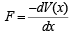As V (x) = constant for x > X0
∴ F = 0 for x > X0
Since F = 0, a = 0

QUESTION: 4

Phase space diagrams are useful tools in analyzing all kinds of dynamical problems. They are especially useful in studying the changes in motion as initial position and momenum are changed.
Here we consider some simple dynamical systems in one dimension. For such systems, phase space is a plane in which position is plotted along horizontal axis and momentum is plotted along vertical axis. The phase space diagram is x(t) vs. p(t) curve in this plane. The arrow on the curve indicates the time flow. For example, the phase space diagram for a particle moving with constant velocity is a straight line as shown in the figure. We use the sign convention in which positon or momentum upwards (or to right) is positive and downwards (or to left) is negative.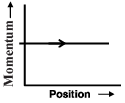Q. The phase space diagram for a ball thrown vertically up from ground is

Solution:

When the ball is thrown upwards, at the point of throw (O) the linear momentum is in upwards direction (and has a maximum value ) and the position is zero. As the time passes, the ball moves upwards and its momentum goes on decreasing and the position becomes positive. The momentum becomes zero at the topmost point (A).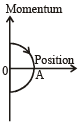As the time increases, the ball starts moving down with an increasing linear momentum in the downward direction (negative) and reaches back to its original position.
These characteristics are represented by graph (d).

QUESTION: 5

Phase space diagrams are useful tools in analyzing all kinds of dynamical problems. They are especially useful in studying the changes in motion as initial position and momenum are changed.
Here we consider some simple dynamical systems in one dimension. For such systems, phase space is a plane in which position is plotted along horizontal axis and momentum is plotted along vertical axis. The phase space diagram is x(t) vs. p(t) curve in this plane. The arrow on the curve indicates the time flow. For example, the phase space diagram for a particle moving with constant velocity is a straight line as shown in the figure. We use the sign convention in which positon or momentum upwards (or to right) is positive and downwards (or to left) is negative.Q. The phase space diagram for simple harmonic motion is a circle centered at the origin. In the figure, the two circles represent the same oscillator but for different initial conditions, and E1 and E2 are the total mechanical energies respectively. Then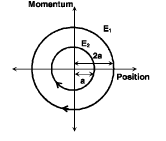Solution:

We know that the total mechanical energy ∝ (Amplitude)2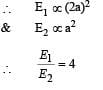QUESTION: 6

Phase space diagrams are useful tools in analyzing all kinds of dynamical problems. They are especially useful in studying the changes in motion as initial position and momenum are changed.
Here we consider some simple dynamical systems in one dimension. For such systems, phase space is a plane in which position is plotted along horizontal axis and momentum is plotted along vertical axis. The phase space diagram is x(t) vs. p(t) curve in this plane. The arrow on the curve indicates the time flow. For example, the phase space diagram for a particle moving with constant velocity is a straight line as shown in the figure. We use the sign convention in which positon or momentum upwards (or to right) is positive and downwards (or to left) is negative.Q. Consider the spring-mass system, with the mass submerged in water, as shown in the figure. The phase space diagram for one cycle of this system isSolution:

As the mass is osicillating in water its amplitude will go on decreasing and the amplitude will decrease with time. Options (c) and (d) cannot be true.
When the position of the mass is at one extreme end in the positive side (the topmost point), the momentum is zero. As the mass moves towards the mean position the momentum increases in the negative direction.
These characteristics are depicted in option (b).

QUESTION: 7

In a simple harmonic oscillator, at the mean position

Solution: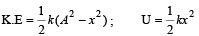At the mean position x = 0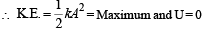QUESTION: 8

If a spring has time period T, and is cut into n equal parts, then the time period of each part will be

Solution:

Let the spring constant of the original spring be k.
Then its time period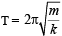where m is the mass of oscillating body.
When the spring is cut into n equal parts, the spring constant of one part becomes nk. Therefore the new time period,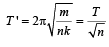QUESTION: 9

A child swinging on a swing in sitting position, stands up, then the time period of the swing will

Solution:

Let the spring constant of the original spring be k. Then its time period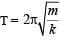where m is the mass of
oscillating body.
When the spring is cut into n equal parts, the spring constant of one part becomes nk. Therefore the new time period,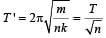QUESTION: 10

A mass M is suspended from a spring of negligible mass. The spring is pulled a little and then released so that the mass executes SHM of  time period T. If the mass is increased by m, the time period becomes 5T/3.  Then the ratio of  m/M is

Solution: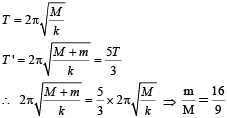QUESTION: 11

Two particles A and B of equal masses are suspended from two massless springs of spring of spring constant k1 and k2, respectively. If the maximum velocities, during oscillation, are equal, the ratio of amplitude of  A and B is

Solution:

Maximum velocity during SHM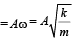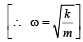Here the maximum velocity is same and m is also same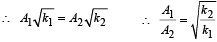QUESTION: 12

The length of a simple pendulum executing simple harmonic motion is increased by 21%. The percentage increase in the time period of the pendulum of  increased length is

Solution: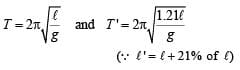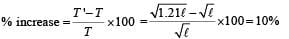QUESTION: 13

The displacement of a particle varies according to the relation x = 4(cos πt + sinπt). The amplitude of the particle is

Solution: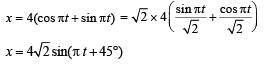QUESTION: 14

A body executes simple harmonic motion. The potential energy (P.E), the kinetic energy (K.E) and total energy (T.E) are measured as a function of displacement x. Which of the following statements is true ?

Solution: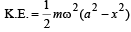When x = 0,  K.E is maximum and is equal to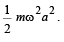QUESTION: 15

The bob of a simple pendulum executes simple harmonic motion in water with a period t, while the period of oscillation of the bob is t0 in air. Neglecting frictional force of water and given that the density of the bob is (4 / 3) x 1000 kg/m3 .
What relationship between t and t0 is true

Solution: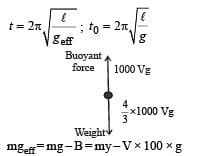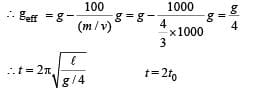QUESTION: 16

A particle at the end of a spring executes S.H.M with a period t1. while the corresponding period for another spring is t2. If the period of oscillation with the two springs in series is T then

Solution:

For first spring,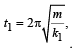For second spring,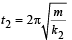when springs are in series then,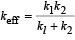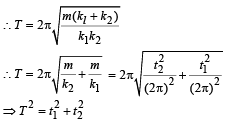where x is the displacement from the mean position

QUESTION: 17

The total energy of a particle, executing simple harmonic motion is where x is the displacement from the mean position, hence total energy is independent of x.

Solution:

At any instant the total  energy is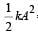= constant,   where  A = amplitude
hence total energy is independent of x.

QUESTION: 18

A particle of mass m is attached to a spring (of spring constant k) and has a natural angular frequency ω0. An external force F(t) pr opor tion al to cos ωt (ω ≠ ω0) is applied to th e oscillator. The time displacement of the oscillator will be proportional to

Solution:

Equation of displacement is given by

x = A sin(ωt +φ)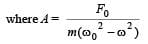QUESTION: 19

In forced oscillation of a particle the amplitude is maximum for a frequency ω1 of the force while the energy is maximum for a frequency ω2 of the force; then

Solution:

The maximum of amplitude and energy is obtained when the frequency is equal to the natural frequency (resonance condition)
∴ w1 = w2

QUESTION: 20

Two simple harmonic motions are represented by the equations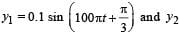= 0.1 cos πt . The phase difference of the velocity of particle 1 with respect to the velocity of particle 2 is

Solution: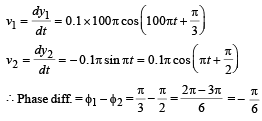QUESTION: 21

The function sin2 (ωt) represents

Solution: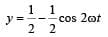QUESTION: 22

The bob of a simple pendulum is a spherical hollow ball filled with water. A plugged hole near the bottom of the oscillating bob gets suddenly unplugged. During observation, till water is coming out, the time period of oscillation would

Solution:

Centre of mass of combination of liquid and hollow portion (at position ℓ), first goes down (to ℓ + Δℓ) and when total water is drained out, centre of mass regain its original position (to ℓ),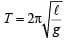∴ ‘T’ first increases and then decreases to original value.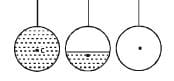QUESTION: 23

If a simple harmonic motion is represented by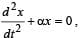its time period is

Solution: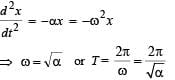QUESTION: 24

Th e maximum velocity of a particle, executin g simple harmonic motion with an amplitude 7 mm, is 4.4 m/s. The period of oscillation is

Solution:

Maximum velocity,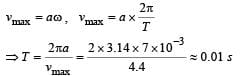QUESTION: 25

Starting from the origin a body oscillates simple harmonically with a period of 2 s. After what time will its kinetic energy be 75% of the total energy?

Solution:

K.E. of a body undergoing SHM is given by,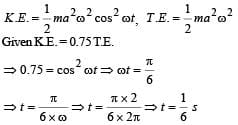QUESTION: 26

Two springs, of force constants k1 and k2 are connected to a mass m as shown. The frequency of oscillation of the mass is f. If both k1 and k2 are made four times their original values, the frequency of oscillation becomes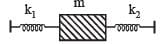Solution:

The two springs are in parallel.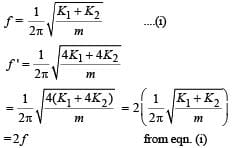QUESTION: 27

A particle of mass m executes simple harmonic motion with amplitude a and frequency v. The average kinetic energy during its motion from the position of equilibrium to the end is

Solution:

(b) KEY CONCEPT : The instantaneous kinetic energy of a particle executing S.H.M. is given by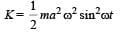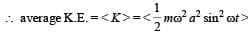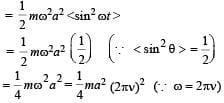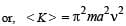QUESTION: 28

The displacement of an object attached to a spring and executing simple harmonic motion is given by x = 2 × 10–2 cos πt metre.The time at which the maximum speed first occurs is

Solution: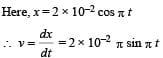For the first time, the speed to be maximum,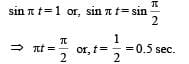QUESTION: 29

A point mass oscillates along the x-axis according to the law x = x0 cos(ωt -π / 4) . If the acceleration of the particle is written as a = A cos(wt +δ) ,then

Solution:

Here,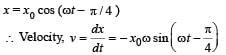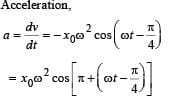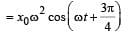...(1)
Acceleration, a = A cos (ωt + δ) ...(2)
Comparing the two equations, we get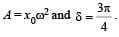QUESTION: 30

If x, v and a denote the displacement, the velocity and the acceleration of a particle executing simple harmonic motion of time period T, then, which of the following does not change with time?

Solution:

For an SHM, the acceleration a = -ω2x where ω2 is a constant. Therefore a/x  is a constant. The time period
T is also constant. Therefor aT/x is a constant.

QUESTION: 31

Two particles are executing simple harmonic motion of the same amplitude A and frequency ω along the x-axis. Their mean position is separated by distance X0(X0 > A). If the maximum separation between them is (X0 + A), the phase difference between their motion is:

Solution:

For X0 + A to be the maximum seperation y one body is at the mean position, the other should be at the extreme.

QUESTION: 32

A mass M, attached to a horizontal spring, executes S.H.M. with amplitude A1. When the mass M passes through its mean position then a smaller mass m is placed over it and both of them move together with amplitude A2. The ratio of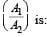Solution:

The net force becomes zero at the mean point.
Therefore, linear momentum must be conserved.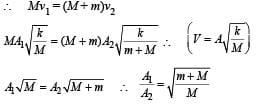QUESTION: 33

If a simple pendulum has significant amplitude (up to a factor of 1/e of original) only in the period between t = 0s to t = τ s, then τ may be called the average life of the pendulum.
When the spherical bob of the pendulum suffers a retardation (due to viscous drag) proportional to its velocity with b as the constant of proportionality, the average life time of the pendulum is (assuming damping is small) in seconds :

Solution:

The equation of motion for the pendulum, suffering retardation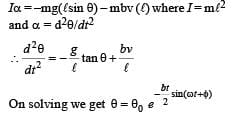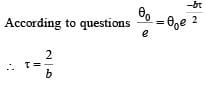QUESTION: 34

The amplitude of a damped oscillator decreases to 0.9 times its original magnitude in 5s. In another 10s it will decrease to a times its original magnitude, where α equals

Solution: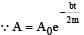(where, A0  = maximum amplitude)
According to the questions, after 5 second,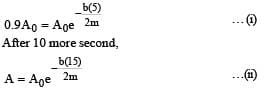From eqns (i) and (ii)
A = 0.729 A0 ∴ α = 0.729

QUESTION: 35

An ideal gas enclosed in a vertical cylindrical container supports a freely moving piston of mass M. The piston and the cylinder have equal cross sectional area A. When the piston is in equilibrium, the volume of the gas is V0 and its pressure is P0. The piston is slightly displaced from the equilibrium position and released. Assuming that the system is completely isolated from its surrounding, the piston executes a simple harmonic motion with frequency

Solution: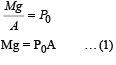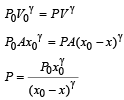Let piston is displaced by distance x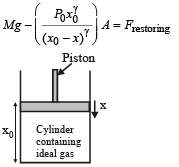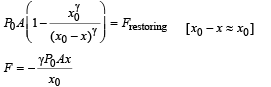∴  Frequency with which piston executes SHM.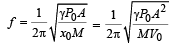QUESTION: 36

A particle moves with simple harmonic motion in a straight line. In first τ s, after starting from rest it travels a distance a, and in next τ s it travels 2a, in same direction, then:

Solution:

In simple harmonic motion, starting from rest,

At t = 0 , x = A
x = Acosωt ..... (i)
When t = τ , x = A – a
When t = 2 τ , x = A –3a
From equation (i)
A – a = Acosω τ ......(ii)
A – 3a = A cos2ω τ ......(iii)
As cos2ωτ = 2 cos2 ωτ – 1 …(iv)

From equation (ii), (iii)  and (iv)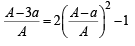|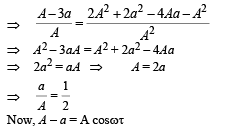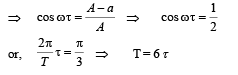QUESTION: 37

A pendulum made of a uniform wire of cross sectional area A has time period T. When an additional mass M is added to its bob, the time period changes to TM. If the Young's modulus of the material of the wire is Y then 1/Y is equal to :

(g = gravitational acceleration)

Solution:

As we know, time period,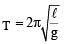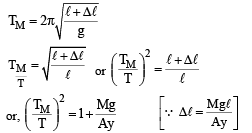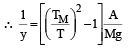QUESTION: 38

For a simple pendulum, a graph is plotted between its kinetic energy (KE) and potential energy (PE) against its displacement d.
Which one of the following represents these correctly? (graphs are schematic and not drawn to scale)

Solution: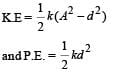At mean position  d = 0. At extremes positions d = A

QUESTION: 39

A particle performs simple harmonic mition with amplitude A. Its speed is trebled at the instant that it is at a distance 2A/3 from equilibrium position. The new amplitude of themotion is :

Solution: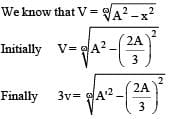Where A'= final amplitude (Given at x = 2A/3, velocity
to trebled)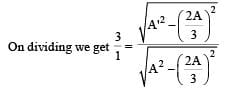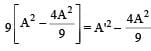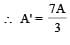Use Code STAYHOME200 and get INR 200 additional OFF Use Coupon Code